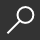menu

## Daily Math Practice, Grade 5 by Evan-Moor Educational Publishers

Overview -
Your grade 5 students will build mastery and retention by applying these lessons for as little as 15 minutes a day. Practice computation, word problems, and dozens of other important math skills are covered, all in a range of difficulty appropriate for grade 5. There are 36 weeks worth of lessons, each divided as follows. Practice five items a day Monday through Thursday: two computation problems, two items that practice a variety of math skills, one word problem. Friday's lesson contains a single problem that is more extensive and may require multiple steps. These problems emphasize reasoning and communication in mathematics. Daily Math Practice, Grade 5 includes practice lessons on the following topics: Computation, multi-digit, addition with and without regrouping, multi-digit subtraction with and without regrouping, multi-digit, multiplication and division, addition, subtraction, multiplication, division of decimals & fractions. Number base ten system, word/standard forms, place value, rounding, estimation, properties/ relationships, factors & GCF, multiples & LCM, equalities/ inequalities, decimals, fractions, percents, integers, Patterns/ Algebra figural patterns, numerical patterns, expressions, function tables, equations, Geometry/ Spatial, 2-dimentional shapes, 3-dimentional shapes, symmetry, congruency, spatial, angle, Measurement, weight, capacity, time, temperature, length, perimeter, area, volume, money, Data/Probability, coordinate graphing constructing graphs, interpreting graphs, range, mode, median, mean, probability,permutations/combination. The page for each day features a place for the student to enter his or her name. All pages are reproducible. Just one example of an exercise found in this book is: "Jorge is thirty years younger than his mom. His mom is two years older than Jorge's dad. If Jorge's dad is thirty-nine, how old is Jorge?". There is an answer key for each week and a scope and sequence chart. The scope and sequence charts detail the specific skills to be practiced and show when they will be presented. The skills included are those found in math texts at each level. Lessons are illustrated with basic line art of things familiar to any fifth grade student. All 112 reproducible pages perforated for easy removal.

Read Full Product Description

Paperback - Teachers Ed.
• \$22.99
Add to Cart
+ Add to Wishlist
local_shippingFor DeliveryIn Stock.
FREE Shipping for Club Members help

storeBuy Online Pickup At StoreNew & Used Marketplace 25 copies from \$5.38

## More About Daily Math Practice, Grade 5 by Evan-Moor Educational Publishers

### Overview

Your grade 5 students will build mastery and retention by applying these lessons for as little as 15 minutes a day. Practice computation, word problems, and dozens of other important math skills are covered, all in a range of difficulty appropriate for grade 5. There are 36 weeks worth of lessons, each divided as follows. Practice five items a day Monday through Thursday: two computation problems, two items that practice a variety of math skills, one word problem. Friday's lesson contains a single problem that is more extensive and may require multiple steps. These problems emphasize reasoning and communication in mathematics. Daily Math Practice, Grade 5 includes practice lessons on the following topics: Computation, multi-digit, addition with and without regrouping, multi-digit subtraction with and without regrouping, multi-digit, multiplication and division, addition, subtraction, multiplication, division of decimals & fractions. Number base ten system, word/standard forms, place value, rounding, estimation, properties/ relationships, factors & GCF, multiples & LCM, equalities/ inequalities, decimals, fractions, percents, integers, Patterns/ Algebra figural patterns, numerical patterns, expressions, function tables, equations, Geometry/ Spatial, 2-dimentional shapes, 3-dimentional shapes, symmetry, congruency, spatial, angle, Measurement, weight, capacity, time, temperature, length, perimeter, area, volume, money, Data/Probability, coordinate graphing constructing graphs, interpreting graphs, range, mode, median, mean, probability,permutations/combination. The page for each day features a place for the student to enter his or her name. All pages are reproducible. Just one example of an exercise found in this book is: "Jorge is thirty years younger than his mom. His mom is two years older than Jorge's dad. If Jorge's dad is thirty-nine, how old is Jorge?". There is an answer key for each week and a scope and sequence chart. The scope and sequence charts detail the specific skills to be practiced and show when they will be presented. The skills included are those found in math texts at each level. Lessons are illustrated with basic line art of things familiar to any fifth grade student. All 112 reproducible pages perforated for easy removal.

### Details

• ISBN-13: 9781557997456
• ISBN-10: 1557997454
• Publisher: Evan Moor Educational Publishers
• Publish Date: January 2014
• Page Count: 128
• Reading Level: Ages 10-11
• Dimensions: 11 x 8.42 x 0.4 inches
• Shipping Weight: 0.82 pounds

Series: Daily Math PracticeRelated Categories